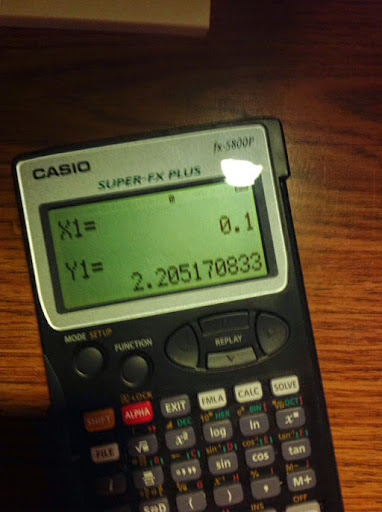## Monday, January 5, 2015

### fx-5800p: Runge-Kutta 4th Order Method - Differential Equations

Runge-Kutta 4th Order Method - Differential Equations

The program RK4 estimates solutions to the differential equation y dy/dx = f(x,y) given the initial condition (x_n, y_n). With step size h, the point (x_n+1, y_n+1) is calculated by:

x_n+1 = x_n + h
y_n+1 = y_n + (k1 + 2*k2 + 2*k3 + k4)/6

Where:

k1 = h * f(x_n, y_n)
k2 = h * f(x_n + h/2, y_n + k1/2)
k3 = h * f(x_n + h/2, y_n + k2/2)
k4 = h * f(x_n + h, y_n + k3)

The program RK4 uses a subroutine FXY. The program FXY is where the y'(x) is stored in terms of X and Y. The result is stored in the variable Z.

Variables used in RK4 and FXY:
A = x_n
B = y_n
C = x_n+1
D = y_n+1
H = step
K = k1
L = k2
M = k3
N = k4
E, X, Y, and Z are also used

Remember for the fx-5800p, all variables (A-Z, and Z[#]s) are global, meaning they will carry over from programs and subroutines.

Anything following the double slash (//) is a comment.

Once the first point (x_n+1, y_n+1) is calculated, RK4 will ask if you want the next point (x_n+2, y_n+2) calculated. Enter 1 at the prompt for "Yes".

Program FXY
type f(X,Y) here → Z

// A RETURN command is implicit.

Program RX4
"INITIAL COND."
"X0"?→ A
"Y0"?→ B
"STEP"?→ H
Lbl 0 // main routine
A → X // k1
B → Y
Prog "FXY"
H * Z → K
A + H ÷ 2 → X // k2
B + K ÷ 2 → Y
Prog "FXY"
H * Z → L
B + L ÷ 2 → Y // k3
Prog "FXY"
H * Z → M
A + H → X // k4
B + M → Y
Prog "FXY"
H * Z → N
X → C // x_n+1
B + (K + 2*L + 2*M + N) ÷ 6 → D // y_n+1
"RESULT"
"X1 = "
C ◢
"Y1 = "
D ◢
"NEXT POINT?"
"1 = YES 0 = NO"
? → E
E ≠ 1 ⇒ Goto E
C → A
D → B
Goto 0
Lbl E
"DONE"

Example: y'(x) = y - x with the initial condition (0,2).
Find y when x = 0.1 and x = 0.2, respectively.
In this case, our step is h = 0.1.

Program FXY will have this:
Y - X → Z

Running RK4 gives these results:
x = 0.1, y = 2.205170833
x = 0.2, y = 2.421402571

Eddie

This blog is property of Edward Shore. 2015